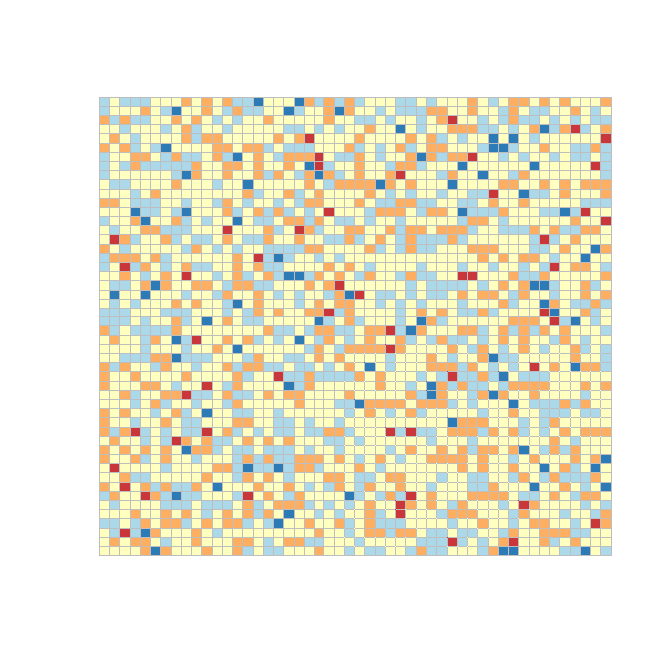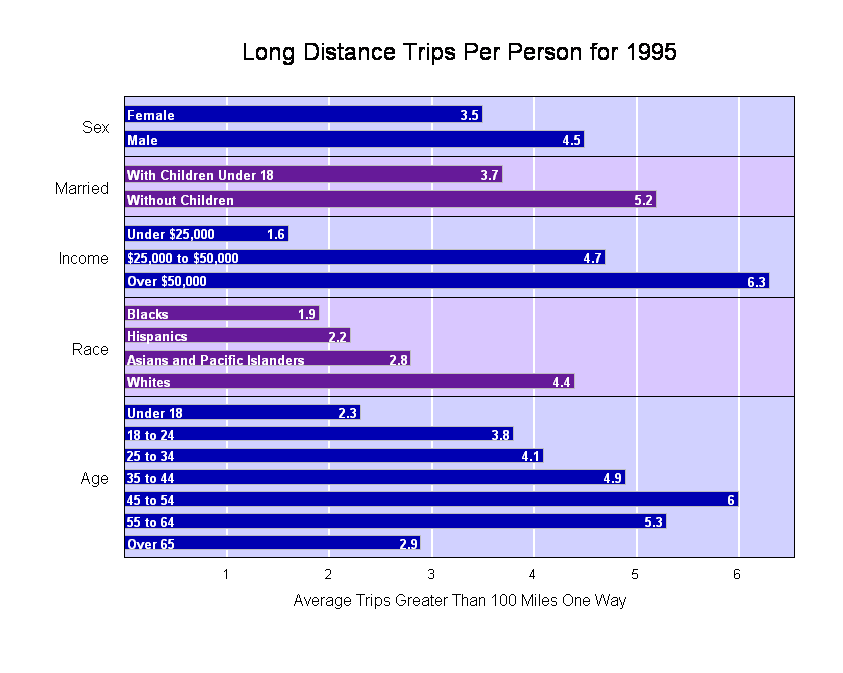Mike Livermore – CSI 773 – Week 2 Assignment – Functions and bar plots - September 2010

2:

coloredMatrix = function(mat,nbreaks=5,colors,

# create a matrix of integer with values from 1 to nbreaks

# This use nbreaks equally space intevals over the range of the data

matColorSubs = cut(mat,breaks=nbreaks,include.lowest=T)

# Define colors if not supplied in the function argument

#     The ones below are diverging colors from ColorBrewer

if(missing(colors)){

colors=c("#2C7BB6","#ABD9E9","#FFFFBF","#FDAE61","#CA373B")

}

# We generate x and y coordinates for the centers of the rectangles

# Start with coordinates yloc and xloc of a single row and a single column

nr = nrow(mat)

nc = ncol(mat)

yloc = nr:1     # Most people think of the top row as row 1

xloc = 1:nc

#  Produce all pairing of one element for each of the two vectors

xyLat = expand.grid(list(y=yloc,x=xloc))

# Extract the vectors in the list as separate vectors

y=xyLat\$y

x=xyLat\$x

# Set of the plot scale

xlab="",ylab="",main=title,cex=1.2)

# Set the size of the rectangles relative to the grid and plot.

# The rectangle centers at integer values

# rect needs the lower left x and y and the upper right x and y

# For x-dx and y-dy  to x+dx and y+dy what should dx and dy

# be for the rectangles to touch?

dx = .5  # This is a single value that will replicate when used below

dy = .5  # This is a single value that will replicate when used below

rect(x-dx,y-dy,x+dx,y+dy,border=border,col=colors[matColorSubs])

return("Done")

}

## End

windows()

mat = matrix(rnorm(2500),ncol=50)

coloredMatrix(mat,border="gray")3: